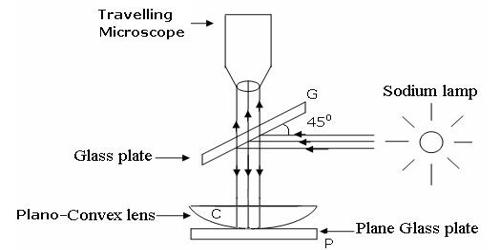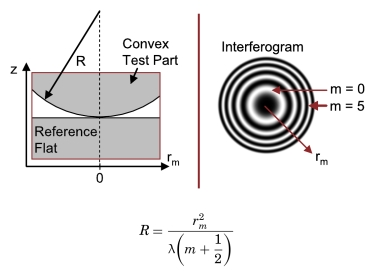# Explain Newton’s Rings Theory

Newton’s Rings Theory

In 1717, Sir Isaac Newton studied the pattern of the ring generated due to the interference of light. These rings are known as Newton’s rings. An important application of interference in thin films is the formation of Newton’s rings.

The formation of Newton’s rings can be explained on the basis of interference between waves which are partially reflected from the top and bottom surfaces of the air film. If t is the thickness of the air film at a point on the film, the refracted wavelet from the lens has to travel a distance t into the film and after reflection from the top surface of the glass plate has to travel the same distance back to reach the point again.

Theory – The formation of Newton’s rings can be explained on the basis of interference between waves which are partially reflected from the top and bottom surfaces of the air film. If t is the thickness of the air film at a point on the film, the refracted wavelet from the lens has to travel a distance t into the film and after reflection from the top surface of the glass plate, has to travel the same distance back to reach the point again.Thus, it travels a total path 2t. One of the two reflections takes place at the surface of the denser medium and hence it introduces an additional phase change of π or an equivalent path difference λ/2 between two wavelets.

∴ The condition for brightness is,

Path difference, δ = 2t + λ/2 = nλ

2t = (2n–1) λ/2

where n = 1, 2, 3 … and λ is the wavelength of light used.

The condition for darkness is, path difference δ = 2t + λ/2 = (2n+1) λ/2

2t = nλ

where n = 0, 1, 2, 3 ….

The thickness of the air film at the point of contact of lens L with glass plate P is zero. Hence, there is no path difference between the interfering waves. So, it should appear bright. But the wave reflected from the denser glass plate has suffered a phase change of π while the wave reflected at the spherical surface of the lens has not suffered any phase change. Hence the point O appears dark. Around the point of contact alternate bright and dark rings are formed.

Rings are fringes of equal thickness. They are observed when light is reflected from a plano-convex lens of a long focal length placed in contact with a plane glass plate.  Newton’s ring pattern is a result of interference between the partially reflected and partially transmitted rays from the lower curved surface of the plano-convex lens and the upper surface of the plane glass plate. When a plano-convex lens of the long focal length is placed over an optically plane glass plate, a thin air film with varying thickness is enclosed between them. When viewed with a monochromatic source, it appears as a series of concentric, alternately bright and dark rings centered at the point of contact between the two surfaces. When the air film is illuminated by monochromatic light normally, alternately bright and dark concentric circular rings are formed with a dark spot at the center. These rings appear in the field of view of the telescope. The wavelength of the monochromatic light and the refractive index of a given transparent liquid medium present in the wedge-shaped film can be calculated by studying the ring pattern. These circular fringes were discovered by Newton and are called Newton’s rings.